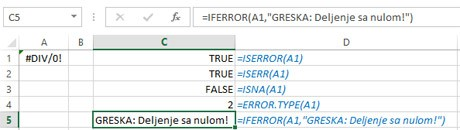# Error checking functions

Errors are happening and this is inevitability! When we know in advance that there is a high likelihood that they can happen, we can use error detection functions. They can discover whether an error has occurred, to return its code, and to show an alternative value instead of a mistake. This can be, for example, a notification about a predefined text about an error or an empty cell, if we want to ignore the error …

The ISERROR, ISERR and ISNA functions are used to find errors. The ISERROR function finds all the errors, ISERR finds all the errors except when the requested value is not found, and the ISNA function finds only an error when the required value is missing. Their syntax:

ISERROR (<formula>)

ISERR (<formula>)

ISNA (<formula>)

The argument of a function can be a formula, function, or cell address where the formula is located.

If an error has already occurred, we can use the ERROR.TYPE function to determine the regular error number. The syntax of this function is:

ERROR.TYPE (<formula>)

The IFERROR function is used to “intercept” errors. It has an argument address of a cell in which an error can arise, as well as an outcome (value, address) if an error occurs. The function syntax is:

IFERROR (<formula>,<outcome>)

The function argument may be the formula, function or address of the cell where the formula is located. The output can also be a formula, a function, an address, or a value that a function returns if an error occurs. Let’s show how this function works on an example. If we enter the formula:

=IFERROR(A1/0,”Error, division by zero”)

the value of “Error, division by zero” will appear in the cell, because the formula we have listed as an argument contains an error.